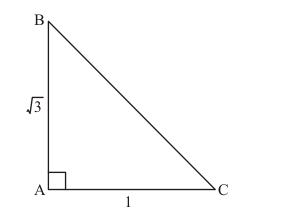# In a ∆ABC, right angled at A,

Question:

In a $\Delta \mathrm{ABC}$, right angled at $\mathrm{A}$, if $\tan \mathrm{C}=\sqrt{3}$, find the value of $\sin \mathrm{B} \cos \mathrm{C}+\cos \mathrm{B} \sin \mathrm{C}$.

Solution:

Given:

$\tan C=\sqrt{3}$

To find: $\sin B \cos C+\cos B \sin C$

The given $\triangle A B C$ is as shown in figure belowSide BC is unknown and can be found using Pythagoras theorem

Therefore,

$B C^{2}=A B^{2}+A C^{2}$

Now by substituting the value of known sides from figure (a)

We get,

$B C^{2}=(\sqrt{3})^{2}+1^{2}$

$=3+1$

$=4$

Now by taking square root on both sides

We get,

$B C=\sqrt{4}$

$=2$

Therefore Hypotenuse side BC = 2 …… (1)

Now $\sin B=\frac{\text { Perpendicular side opposite to } \angle B}{\text { Hypotenuse }}$

Therefore,

$\sin B=\frac{A C}{B C}$

Now by substituting the values from equation (1) and figure (a)

We get,

$\sin B=\frac{1}{2}$.....(2)

Now $\cos B=\frac{\text { Base side adjacent to } \angle B}{\text { Hypotenuse }}$

Therefore,

$\cos B=\frac{A B}{B C}$

Now by substituting the values from equation (1) and figure (a)

We get,

$\cos B=\frac{\sqrt{3}}{2}$....(3)

Now $\sin C=\frac{\text { Perpendicular side opposite to } \angle C}{\text { Hypotenuse }}$

Therefore,

$\sin C=\frac{A B}{B C}$

Now by substituting the values from equation (1) and figure (a)

We get,

$\sin C=\frac{\sqrt{3}}{2} \ldots \ldots$(4)

Now by definition,

$\tan C=\frac{\sin C}{\cos C}$

Therefore,

$\cos C=\frac{\sin C}{\tan C}$

Now by substituting the value of $\sin C$ and $\tan C$ from equation (4) and given data respectively

We get,

$\cos C=\frac{\frac{\sqrt{3}}{2}}{\sqrt{3}}$

$\cos C=\frac{\sqrt{3}}{2 \sqrt{3}}$

Now $\sqrt{3}$ gets cancelled as it is present in both numerator and denominator

Therefore,

$\cos C=\frac{1}{2}$.....(5)

Now by substituting the value of $\sin B, \cos B, \sin C$ and $\cos C$ from equation $(2),(3),(4)$ and (5) respectively in $\sin B \cos C+\cos B \sin C$

We get,

$\sin B \cos C+\cos B \sin C=\frac{1}{2} \times \frac{1}{2}+\frac{\sqrt{3}}{2} \times \frac{\sqrt{3}}{2}$

$=\frac{1}{4}+\frac{3}{4}$

$=\frac{4}{4}$

$=1$

$\sin B \cos C+\cos B \sin C=1$